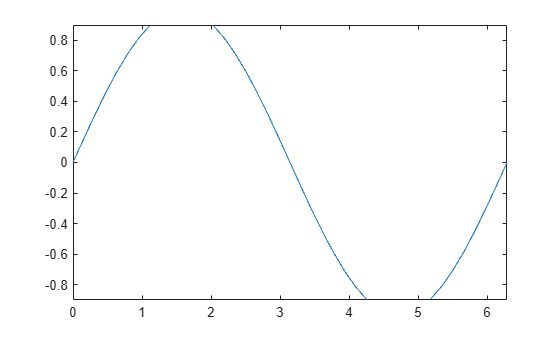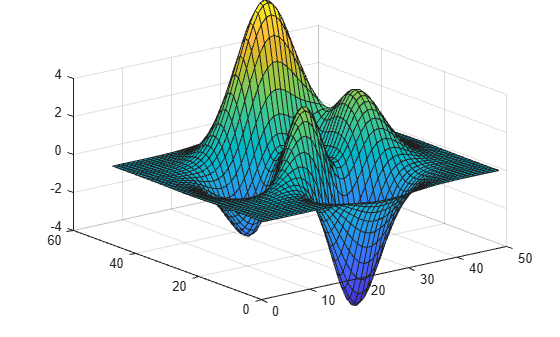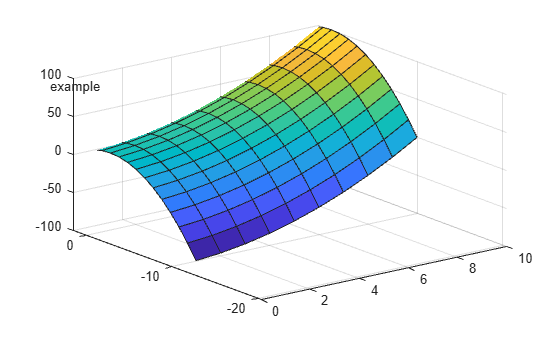Main Content

# Clipping in Plots and Graphs

This example shows how MATLAB® uses clipping in plots and how to control clipping.

### What is Clipping?

Clipping occurs when part of a plot occurs outside the boundaries of an axes. In MATLAB®, the part of the plot that is clipped does not appear on the screen or in printed output. The axis limits of the plot determine the boundaries.

### Turn Clipping Off

By default, MATLAB clips plots that extend outside of the axes limits.

```figure surf(peaks) zlim([-4 4])```Use the axes `Clipping` property to control the clipping behavior.

```ax = gca; % get the current axis ax.Clipping = 'off'; % turn clipping off```### Control the Clipping Style

Use the `ClippingStyle` property to control the way clipping works. If the `ClippingStyle` is set to `'3dbox'`, then MATLAB clips the plots to the volume defined by the limits of the x, y, and z axes. If the `ClippingStyle` is set to `'rectangle'`, then MATLAB clips the plots to an imaginary rectangle drawn around the outside of the x, y, and z axes. The plots below show the difference between the two clipping styles.### Clipping in 2D plots

Clipping is also used in 2D plots. For example, MATLAB clips the sine wave in the plot below.

```x = -pi:pi/20:pi; y = sin(-pi:pi/20:pi); plot(x,y) ylim([-0.9 0.9])```If clipping is turned off, then MATLAB displays the entire sine wave.

```ax = gca; ax.Clipping = 'off';```### Clipping and Markers

Clipping does not affect markers drawn at each data point as long as the data point itself is inside the x and y axis limits of the plot. MATLAB displays the entire marker even if it extends slightly outside the boundaries of the axes.

```p = plot(1:10,'*'); p.MarkerSize = 10; axis([1 10 1 10])```Download ebook